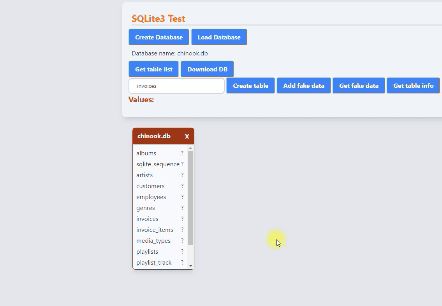I continue with my exploration of SQLite 3 for browsers. That is, the JavaScript version that allows you to use code compiled in WebAssembly. A few days ago I posted my notes on how to create a database, add tables and data, and how to extract data from a table. Today I report my notes on how to read a database saved locally, how to obtain the list of tables present and, finally, how to understand which are the columns of a table.### How to open a database

To open a database I need a way to pass it to the web page. The easiest way is to do this using an element `input` with `type="file"`.

``````<input type="file" bind:files />
``````

This way, when the user selects a file, its contents are saved in a variable `files` which is an array of `File` (see `Files` ).

``````\$: if (files) {
const file = files;
console.log(file.name, file.size);
}
``````

But I need a function to read the contents of the file. I create a specific function, `readDatabase`:

``````const readDatabase = (file) => {
const arrayBuffer = this.result as ArrayBuffer;
let bytes = new Uint8Array(arrayBuffer);
const p = sqlite3.wasm.allocFromTypedArray(bytes);
const db = new sqlite3.oo1.DB();
let rc = sqlite3.capi.sqlite3_deserialize(
db.pointer,
"main",
p,
bytes.length,
bytes.length,
0
);
const dbName = file.name;
return db;
});
};
``````

Going to break down the code, I need an event that intercepts the file and reads it as an ArrayBuffer:

``````const readAsArrayBuffer = (file) => {
return new Promise((resolve, reject) => {
});
};
``````

After I read the file I can convert it to a byte array:

``````const arrayBufferToBytes = (arrayBuffer) => {
return new Uint8Array(arrayBuffer);
};
``````

This way I can use the sqlite3_deserialize method:

``````const deserialize = (db, bytes) => {
const p = sqlite3.wasm.allocFromTypedArray(bytes);
let rc = sqlite3.capi.sqlite3_deserialize(
db.pointer,
"main",
p,
bytes.length,
bytes.length,
sqlite3.capi.SQLITE_DESERIALIZE_FREEONCLOSE |
sqlite3.capi.SQLITE_DESERIALIZE_RESIZEABLE
);
return db;
};
``````

So putting the pieces together I can write the function `readDatabase`:

``````const readAsArrayBuffer = (file) => {
return new Promise((resolve, reject) => {
});
};

const readDatabase = (arrayBuffer) => {
const sqlite3 = self["sqlite3"];
let bytes = new Uint8Array(arrayBuffer);
const p = sqlite3.wasm.allocFromTypedArray(bytes);
const db = new sqlite3.oo1.DB();
let rc = sqlite3.capi.sqlite3_deserialize(
db.pointer,
"main",
p,
bytes.length,
bytes.length,
sqlite3.capi.SQLITE_DESERIALIZE_FREEONCLOSE |
sqlite3.capi.SQLITE_DESERIALIZE_RESIZEABLE
);
return db;
};

let db;
let dbName;

\$: if (files) {
dbName = files.name;
files = null;
getTableList();
}).catch((e) => {
console.log(e);
});
}
``````

### Extract the list of tables

Now I can extract the table list. There are two possible ways. The first one, the one I used, is to query the table `sqlite_master` or the table `sqlite_schema`:

``````const getTableList = (db) => {
let listTable = [];
db.exec({
sql: `select name from sqlite_master where type='table'`,
rowMode: "array", // 'array' (default), 'object', or 'stmt'
callback: function (row) {
console.log("row ", ++this.counter, "=", row);
listTable = [...listTable, row];
}.bind({ counter: 0 }),
});
return listTable;
};
``````

I already explained how to use `exec`. I simplify the code by eliminating the `callback` and using `returnValue`. I then add the `flat` method to convert the array of arrays to a plain array:

``````const getTableList = (db) =>
db
.exec({
sql: `select name from sqlite_master where type='table'`,
returnValue: "resultRows",
})
.flat();
``````

### Extract column names from a table

The second way to obtain the list of table names in a database involves the use of PRAGMA Statements. They are nothing more than additions to the SQL syntax that allow you to query the database. For example, the command `PRAGMA table_list` returns the list of tables contained in the database. Instead the command `PRAGMA table_info(table_name)` returns information about the columns of a table. This way I can extract the list of column names of a table:

``````let idInfo = 0;

const getTableInfo = (tableName) => {
let infoTable = [];
idInfo++;
db.exec({
sql: `PRAGMA table_info("\${tableName}")`,
rowMode: "object",
callback: function (row) {
console.log("row ", ++this.counter, "=", JSON.stringify(row));
infoTable = [...infoTable, row];
}.bind({ counter: 0 }),
});
tableInfo = [...tableInfo, { infoTable, tableName, idInfo }];
};
``````

Again, I simplify the code by eliminating the `callback` and using `returnValue`:

``````const getTableColumns = (db, tableName) => [
{
tableName,
id: Math.random(),
columns: db.exec({
sql: `PRAGMA table_info("\${tableName}")`,
rowMode: "object",
returnValue: "resultRows",
}),
},
];
``````

And that’s all for today.

Tags:

Categorie:

Aggiornato: